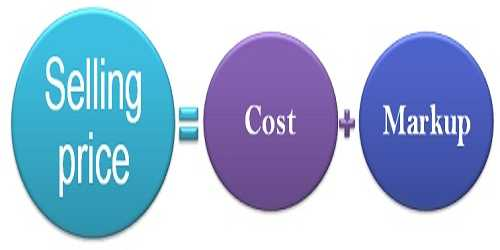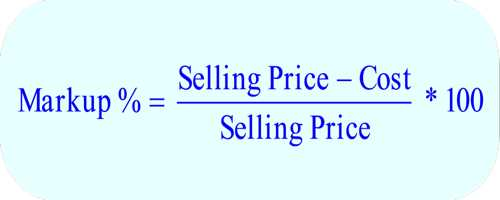Marketing

# MarkupMarkup is the amount added to the cost price of goods to cover overheads and profit. Stores buy items from a wholesaler or distributor and increase the price when they sell the items to consumers. The increase in price provides money for the operation of the store and the salaries of people who work in the store.

Markups occur when dealers act as principals, buying and selling securities from their own accounts at their own risk, as opposed to brokers receiving a fee for facilitating a transaction. A store may have a rule that the price of a certain type of item needs to be increased by a certain percentage to determine how much to sell it for. This percentage is called the markup.

As an example, a company pays \$5 for widgets. The company wants a 10 percent profit on the goods.

1. Determine the markup the company wants and the cost of the good. In the example, the cost is \$5 and the markup rate is 10 percent.
2. Subtract 1 from the markup rate. In the example, 1 minus 10 percent equals 90 percent or 0.9.
3. Divide the cost of the product by the number calculated in Step 2. In the example, \$5 divided by 0.9 equals \$5.56. So if the company uses a 10 percent markup, it will sell the product for \$5.56.

If the cost is known and the percentage markup is known, the sale price is the original cost plus the amount of markup. For example, if the original cost is \$4.00 and the markup is 25%, the sales price should be \$4.00 + \$4.00*25/100 = \$5.00.

A faster way to calculate the sale price is to make the original cost equal to 100%. The markup is 25% so the sales price is 125% of the original cost. In the example, \$4.00 * 125/100 = \$5.00.Calculation:

The markup sales are expressed as a percentage increase as to try and ensure that a company can receive the proper amount of gross or profit margin. Markups are normally used in retail or wholesale business as it is an easy way to price items when a store contains several different goods.

The markup percentage calculation is cost X markup percentage, and then add that to the original unit cost to arrive at the sales price. The markup equation or markup formula is given below in several different formats. For example, if a product costs \$100, the selling price with a 25% markup would be \$125.

Gross Profit Margin = Sales Price – Unit Cost = \$125 – \$100 = \$25.

Percentage = Gross Profit Margin/Unit Cost = \$25/\$100 = 25%.

Sales Price = Cost X Percentage + Cost = \$100 X 25% + \$100 = \$125.

One of the pitfalls in using the markup percentage to calculate your prices is that it is difficult to ensure that you have taken into consideration all of your costs. By using a simple rule of thumb calculation, you often miss out on indirect costs.

Information Source: